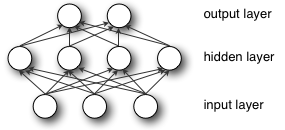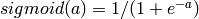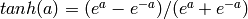Home 数据分析 多层感知机（MLP）原理简介f(W1X+b1)，W1是权重（也叫连接系数），b1是偏置，函数f 可以是常用的sigmoid函数或者tanh函数：# sklearn.neural_network.MLPClassifier

class sklearn.neural_network.MLPClassifier(hidden_layer_sizes=(100)activation=’relu’, solver=’adam’alpha=0.0001batch_size=’auto’learning_rate=’constant’learning_rate_init=0.001power_t=0.5max_iter=200shuffle=Truerandom_state=Nonetol=0.0001verbose=Falsewarm_start=Falsemomentum=0.9nesterovs_momentum=Trueearly_stopping=Falsevalidation_fraction=0.1beta_1=0.9beta_2=0.999epsilon=1e-08n_iter_no_change=10)[source]

Multi-layer Perceptron classifier.

This model optimizes the log-loss function using LBFGS or stochastic gradient descent.

New in version 0.18.

 Parameters: hidden_layer_sizes : tuple, length = n_layers – 2, default (100,) The ith element represents the number of neurons in the ith hidden layer. activation : {‘identity’, ‘logistic’, ‘tanh’, ‘relu’}, default ‘relu’ Activation function for the hidden layer. ‘identity’, no-op activation, useful to implement linear bottleneck, returns f(x) = x ‘logistic’, the logistic sigmoid function, returns f(x) = 1 / (1 + exp(-x)). ‘tanh’, the hyperbolic tan function, returns f(x) = tanh(x). ‘relu’, the rectified linear unit function, returns f(x) = max(0, x) solver : {‘lbfgs’, ‘sgd’, ‘adam’}, default ‘adam’ The solver for weight optimization. ‘lbfgs’ is an optimizer in the family of quasi-Newton methods. ‘sgd’ refers to stochastic gradient descent. ‘adam’ refers to a stochastic gradient-based optimizer proposed by Kingma, Diederik, and Jimmy Ba Note: The default solver ‘adam’ works pretty well on relatively large datasets (with thousands of training samples or more) in terms of both training time and validation score. For small datasets, however, ‘lbfgs’ can converge faster and perform better. alpha : float, optional, default 0.0001 L2 penalty (regularization term) parameter. batch_size : int, optional, default ‘auto’ Size of minibatches for stochastic optimizers. If the solver is ‘lbfgs’, the classifier will not use minibatch. When set to “auto”, batch_size=min(200, n_samples) learning_rate : {‘constant’, ‘invscaling’, ‘adaptive’}, default ‘constant’ Learning rate schedule for weight updates. ‘constant’ is a constant learning rate given by ‘learning_rate_init’. ‘invscaling’ gradually decreases the learning rate learning_rate_ at each time step ‘t’ using an inverse scaling exponent of ‘power_t’. effective_learning_rate = learning_rate_init / pow(t, power_t) ‘adaptive’ keeps the learning rate constant to ‘learning_rate_init’ as long as training loss keeps decreasing. Each time two consecutive epochs fail to decrease training loss by at least tol, or fail to increase validation score by at least tol if ‘early_stopping’ is on, the current learning rate is divided by 5. Only used when solver='sgd'. learning_rate_init : double, optional, default 0.001 The initial learning rate used. It controls the step-size in updating the weights. Only used when solver=’sgd’ or ‘adam’. power_t : double, optional, default 0.5 The exponent for inverse scaling learning rate. It is used in updating effective learning rate when the learning_rate is set to ‘invscaling’. Only used when solver=’sgd’.

## 概述$f(z) = \frac{1}{1+\exp(-z)}.$$f(z) = \tanh(z) = \frac{e^z - e^{-z}}{e^z + e^{-z}},$$\textstyle \tanh(z)$ 函数是sigmoid函数的一种变体，它的取值范围为$\textstyle [-1,1]$ ，而不是sigmoid函数的$\textstyle [0,1]$ 。

## 神经网络模型\begin{align} a_1^{(2)} &= f(W_{11}^{(1)}x_1 + W_{12}^{(1)} x_2 + W_{13}^{(1)} x_3 + b_1^{(1)}) \\ a_2^{(2)} &= f(W_{21}^{(1)}x_1 + W_{22}^{(1)} x_2 + W_{23}^{(1)} x_3 + b_2^{(1)}) \\ a_3^{(2)} &= f(W_{31}^{(1)}x_1 + W_{32}^{(1)} x_2 + W_{33}^{(1)} x_3 + b_3^{(1)}) \\ h_{W,b}(x) &= a_1^{(3)} = f(W_{11}^{(2)}a_1^{(2)} + W_{12}^{(2)} a_2^{(2)} + W_{13}^{(2)} a_3^{(2)} + b_1^{(2)}) \end{align}\begin{align} z^{(2)} &= W^{(1)} x + b^{(1)} \\ a^{(2)} &= f(z^{(2)}) \\ z^{(3)} &= W^{(2)} a^{(2)} + b^{(2)} \\ h_{W,b}(x) &= a^{(3)} = f(z^{(3)}) \end{align}\begin{align} z^{(l+1)} &= W^{(l)} a^{(l)} + b^{(l)} \\ a^{(l+1)} &= f(z^{(l+1)}) \end{align}

———————————————————-

MLP是一个监督学习算法，图1是带一个隐藏层的MLP模型

MLP的优点是：
– 可以学习非线性模型
– 使用partial_fit 实时学习
MLP的缺点是：
– 有隐藏层的MLP包含一个非凸性损失函数，存在超过一个最小值，所以不同的随机初始权重可能导致不同验证精确度
– MLP要求调整一系列超参数，比如隐藏神经元，隐藏层的个数以及迭代的次数
– MLP对特征缩放比较敏感

2.分类
MLPClassifier使用BP算法来实现一个多层感知机。

>>> from sklearn.neural_network import MLPClassifier
>>> X=[[0.,0.],[1.,1.]]
>>> y=[0,1]
>>> clf = MLPClassifier(solver=’lbfgs’,alpha=1e-5,hidden_layer_sizes=(5,2),random_state=1)
>>> clf.fit(X,y)
MLPClassifier(activation=’relu’, alpha=1e-05, batch_size=’auto’, beta_1=0.9,
beta_2=0.999, early_stopping=False, epsilon=1e-08,
hidden_layer_sizes=(5, 2), learning_rate=’constant’,
learning_rate_init=0.001, max_iter=200, momentum=0.9,
nesterovs_momentum=True, power_t=0.5, random_state=1, shuffle=True,
solver=’lbfgs’, tol=0.0001, validation_fraction=0.1, verbose=False,
warm_start=False)
>>> clf.predict([[2.,2.],[-1.,-2.]])
array([1, 0])
>>> [coef.shape for coef in clf.coefs_]
[(2L, 5L), (5L, 2L), (2L, 1L)]
>>> clf.predict_proba([[2.,2.],[1.,2.]])
array([[  1.96718015e-04,   9.99803282e-01],
[  1.96718015e-04,   9.99803282e-01]])

1. hidden_layer_sizes :元祖格式，长度=n_layers-2, 默认(100，），第i个元素表示第i个隐藏层的神经元的个数。
2. activation :{‘identity’, ‘logistic’, ‘tanh’, ‘relu’}, 默认‘relu
– ‘identity’： no-op activation, useful to implement linear bottleneck，

– ‘logistic’：the logistic sigmoid function, returns f(x) = 1 / (1 + exp(-x)).
– ‘tanh’：the hyperbolic tan function, returns f(x) = tanh(x).
– ‘relu’：the rectified linear unit function, returns f(x) = max(0, x)
– lbfgs：quasi-Newton方法的优化器
– sgd：随机梯度下降
– adam： Kingma, Diederik, and Jimmy Ba提出的机遇随机梯度的优化器

5. alpha :float,可选的，默认0.0001,正则化项参数
6. batch_size : int , 可选的，默认‘auto’,随机优化的minibatches的大小，如果solver是‘lbfgs’，分类器将不使用minibatch，当设置成‘auto’，batch_size=min(200,n_samples)
– ‘constant’: 有‘learning_rate_init’给定的恒定学习率
– ‘incscaling’：随着时间t使用’power_t’的逆标度指数不断降低学习率learning_rate_ ，effective_learning_rate = learning_rate_init / pow(t, power_t)
8. max_iter: int，可选，默认200，最大迭代次数。
9. random_state:int 或RandomState，可选，默认None，随机数生成器的状态或种子。
11. tol：float, 可选，默认1e-4，优化的容忍度
13. power_t: double, optional, default 0.5，只有solver=’sgd’时使用，是逆扩展学习率的指数.当learning_rate=’invscaling’，用来更新有效学习率。
14. verbose : bool, optional, default False,是否将过程打印到stdout
15. warm_start : bool, optional, default False,当设置成True，使用之前的解决方法作为初始拟合，否则释放之前的解决方法。
16. momentum : float, default 0.9,Momentum(动量） for gradient descent update. Should be between 0 and 1. Only used when solver=’sgd’.
17. nesterovs_momentum : boolean, default True, Whether to use Nesterov’s momentum. Only used when solver=’sgd’ and momentum > 0.
18. early_stopping : bool, default False,Only effective when solver=’sgd’ or ‘adam’,判断当验证效果不再改善的时候是否终止训练，当为True时，自动选出10%的训练数据用于验证并在两步连续爹迭代改善低于tol时终止训练。
19. validation_fraction : float, optional, default 0.1,用作早期停止验证的预留训练数据集的比例，早0-1之间，只当early_stopping=True有用
20. beta_1 : float, optional, default 0.9，Only used when solver=’adam’，估计一阶矩向量的指数衰减速率，[0,1)之间
21. beta_2 : float, optional, default 0.999,Only used when solver=’adam’估计二阶矩向量的指数衰减速率[0,1)之间
22. epsilon : float, optional, default 1e-8,Only used when solver=’adam’数值稳定值。

– classes_:每个输出的类标签
– loss_:损失函数计算出来的当前损失值
– coefs_:列表中的第i个元素表示i层的权重矩阵
– intercepts_:列表中第i个元素代表i+1层的偏差向量
– n_iter_ ：迭代次数
– n_layers_:层数
– n_outputs_:输出的个数
– out_activation_:输出激活函数的名称。

– fit(X,y):拟合
– get_params([deep]):获取参数
– predict(X):使用MLP进行预测
– predic_log_proba(X):返回对数概率估计
– predic_proba(X)：概率估计
– score(X,y[,sample_weight]):返回给定测试数据和标签上的平均准确度
-set_params(**params):设置参数。
———————

MLP回归案例：

# -*- coding:utf-8 -*-
import matplotlib.pyplot as plt
from sklearn.neural_network import MLPRegressor

x_train = []
y_train = []

for i in range(100):
if i in range(50, 70):
continue
y = 8 * i ** 4 + 7
#y = 8 * i + 7
x_train.append([i])
y_train.append(y)

forestReg=MLPRegressor(solver='lbfgs', hidden_layer_sizes=(2000,), random_state=1)
forestReg.fit(x_train, y_train)

x_test = [[i] for i in range(50, 70)]
print x_test
p = list(forestReg.predict(x_test))
print p

plt.scatter(map(lambda x: x, x_train), y_train, c="green")
plt.scatter(map(lambda x: x, x_test), p, marker="s", c="yellow")
plt.show()# -*- coding:utf-8 -*-

import time
import math
import matplotlib.pyplot as plt
from sklearn.neural_network import MLPRegressor
import copy

x_train = []
y_train = []

for i in range(5000):
if i in range(5000, 5500):
continue
y = 8 * i ** 3 + 7
x_train.append([i])
y_train.append(y)

forestReg=MLPRegressor(solver='lbfgs', max_iter=3000, activation="relu",hidden_layer_sizes=(2000,), random_state=int(time.time()))
forestReg.fit(copy.deepcopy(x_train), copy.deepcopy(y_train))

x_test = [[i] for i in range(5000, 5500)]
print x_test
p = list(forestReg.predict(copy.deepcopy(x_test)))
print p

plt.scatter(map(lambda x: x, x_train), y_train, c="green")
plt.scatter(map(lambda x: x, x_test), p, marker="s", c="yellow")
plt.show()



0 comment

#### You may also like

error: Alert: Content is protected !!